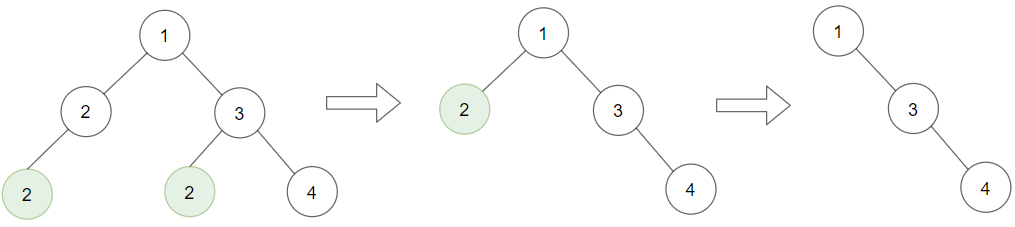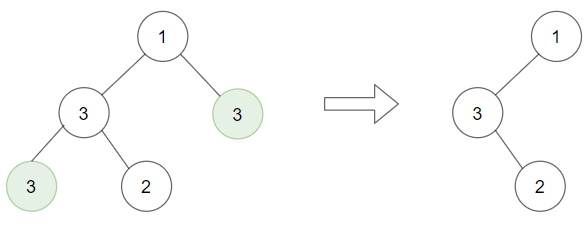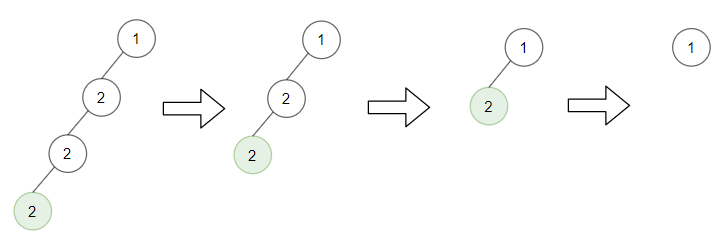1325. Delete Leaves With a Given Value

#### QUESTION:

Given a binary tree root and an integer target, delete all the leaf nodes with value target.

Note that once you delete a leaf node with value target, if its parent node becomes a leaf node and has the value target, it should also be deleted (you need to continue doing that until you cannot).

Example 1:``````Input: root = [1,2,3,2,null,2,4], target = 2
Output: [1,null,3,null,4]
Explanation: Leaf nodes in green with value (target = 2) are removed (Picture in left).
After removing, new nodes become leaf nodes with value (target = 2) (Picture in center).
``````

Example 2:``````Input: root = [1,3,3,3,2], target = 3
Output: [1,3,null,null,2]
``````

Example 3:``````Input: root = [1,2,null,2,null,2], target = 2
Output: 
Explanation: Leaf nodes in green with value (target = 2) are removed at each step.
``````

Constraints:

``````The number of nodes in the tree is in the range [1, 3000].
1 <= Node.val, target <= 1000
``````

#### SOLUTION:

``````class Solution {
func removeLeafNodes(_ root: TreeNode?, _ target: Int) -> TreeNode? {
var result:Bool = removeLeafNodesHelper(root, target)
return result ? nil : root
}

func removeLeafNodesHelper(_ root: TreeNode?, _ target: Int) -> Bool {
if root == nil {
return false
}
var left:Bool = removeLeafNodesHelper(root?.left, target)
var right:Bool = removeLeafNodesHelper(root?.right, target)
if left {
root?.left = nil
}
if right {
root?.right = nil
}
if root?.val == target && root?.left == nil && root?.right == nil {
return true
} else {
return false
}
}
}
``````